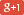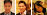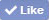# GMAT Math #6 – Isosceles Triangles in Data Sufficiency

Hi Zeke,

I need help with a Question, I have found in the GMAT prep software,

The following is the question:

How do I solve for the answer?
I read somewhere online, that it says that triangles ABO and BCO are isosceles triangles, why so ?GMATPill:
Yes, ABO and BCO are isosceles. However, they are not exactly the same isosceles. OC and OB are both the same length because each is a radius of the semi circle.Since they are the same length, that means BCO is isosceles.

If OC = OB, and we are told that OC = AB, then OB must = AB – which means ABO is isosceles as well.

(1) tells us that COD is 60. We also know AB = OB = OC.

COD forms a 180 straight line with triangle AOC. So AOC + COD = 180.

Note one of the popular formulas commonly tested on GMAT is to equate the sum of the 2 angles inside the triangle to “exterior angle” of the triangle.

In other words, OAC + ACO = COD
Since COD = 60, we know OAC + ACO = 60.
But how do we know the breakdown between OAC and ACO?

Well, since OB = OC, then OBC = OCB = OCA
the part that matters is: OBC = OCA

But wait, notice that OBC is another one of those exterior angles. This time, it’s the exterior angle of triangle BAO. Since BA = BO, the angle BAO = BOA.
And that means the two angles BAO + BOA = exterior OBC
In fact, 2 * BAO = OBC, since BAO = BOA.

Effectively, OBC = 2 * OAB.
Equally, OCA = 2 * OAC

If the ratio of OCA to OAC is 2 to 1 and they both add up to 60, then OCA = 40, an OAC = 20.
But we are asked what BAO is. Well, BAO is the same as OAC, which is 20 degrees.

As such, (1) alone is enough info to say that angle BAO is 20 degrees.

(2) The degree measure of angle BCO is 40.
Well, BCO is the same as OCA mentioned above. If we know OCA = 40 and we know OCA = 2 * OAC, then
40 = 2 * OAC
OAC = 20.
And of course, OAC is the same as BAO.

So (2) alone is also enough information to get the measurement of angle BAO.

Note all of the above may be difficult to understand through text. That’s why we suggest watching our online video course where the explanations are a lot easier to follow in real time.

Hope that helps.

/images/logo.png" />